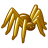# calculating distance (in meters/miles) from point to &quot;eastern&quot; polygon

142
1
04-16-2012 01:37 PMNew Contributor
I am using ArcGIS 10, and trying to do the following:

I have a layer file of points, and I would like to calculate the distance from each point to the nearest "eastern" polygon (U.S. state). I know there are tools for calculating distances from points to polygons (e.g., Near), but I don't know how (or, if you can) specify the direction of the polygon.

In addition, I need the output units to be in meters or miles (not decimal degrees).

Any suggestions?

Tags (3)Regular Contributor II
The Near tool has an option to record the direction angle so you could find the distance to all eastern states.

Select the states to the east of the points could be done by comparing the longitude of the point and the centroid of the state, or  maybe the extent of the state. If you make a list of these they could be input into the Near tool. They might need to be in separate featureclasses, one for each state.

So while you are  there, project the states into projected units  that you require.

At 10.x you could create geometry objects, one for each state, to hold them all in memory instead of 50 featureclasses.

I am trying to think of a way of avoid a loop around each point.

Another approach might be to create a line eastwards from each point, intersect the whole lot at once and find the first intersection from each point.